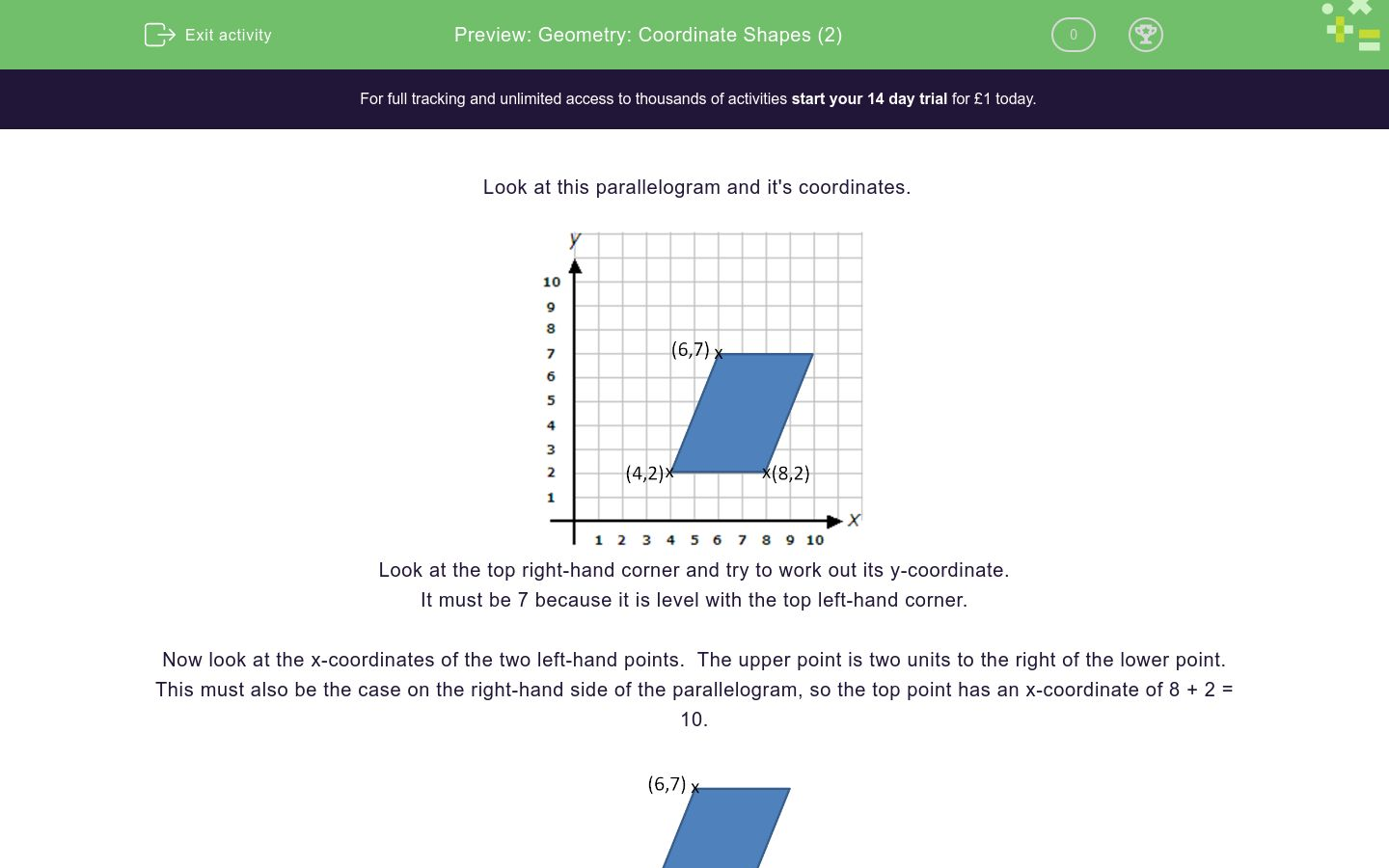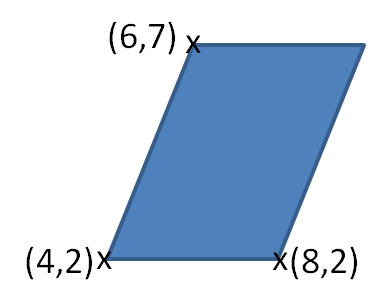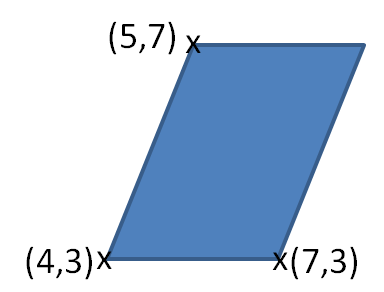# Geometry: Coordinate Shapes (2)

In this worksheet, students work out the coordinates of the missing corner of a shape, given the other three corners.Key stage:  KS 2

Curriculum topic:   Geometry: Position and Direction

Curriculum subtopic:   Know, Describe and Present Shapes

Difficulty level:### QUESTION 1 of 10

Look at this parallelogram and it's coordinates.Look at the top right-hand corner and try to work out its y-coordinate.

It must be 7 because it is level with the top left-hand corner.

Now look at the x-coordinates of the two left-hand points.  The upper point is two units to the right of the lower point.

This must also be the case on the right-hand side of the parallelogram, so the top point has an x-coordinate of 8 + 2 = 10.The top right-hand corner has coordinates (10, 7) and it is has been possible to work this out without reading the axes.

What are the coordinates of the top right-hand corner of this rectangle?(8, 4)

(8, 8)

(2, 8)

(2, 4)

What is the x-coordinate of the top right-hand corner of this square?2

4

6

8

What is the y-coordinate of the top right-hand corner of this square?2

4

6

8

What are the coordinates of the top right-hand corner of this square?(2, 6)

(6, 8)

(8, 6)

(8, 8)

What is the x-coordinate of the top right-hand corner of this rectangle?4

6

7

8

What is the y-coordinate of the top right-hand corner of this rectangle?4

6

7

8

What are the coordinates of the top right-hand corner of this rectangle?(8, 7)

(7, 8)

(7, 7)

(8, 8)

What is the x-coordinate of the top right-hand corner of this parallelogram?6

7

8

9

What is the y-coordinate of the top right-hand corner of this parallelogram?6

7

8

9

What are the coordinates of the top right-hand corner of this parallelogram?(7, 7)

(7, 8)

(8, 8)

(8, 7)

• Question 1

What are the coordinates of the top right-hand corner of this rectangle?(8, 8)
EDDIE SAYS
Because the shape is a rectangle, the bottom right x-coordinate is 8, so the top right x-coordinate must also be 8. The top left y-coordinate is 8, so the top right y-coordinate must also be 8. Answer (8, 8)
• Question 2

What is the x-coordinate of the top right-hand corner of this square?8
EDDIE SAYS
Because the shape is a square, the bottom right x-coordinate is 8, so the top right x-coordinate must also be 8.
• Question 3

What is the y-coordinate of the top right-hand corner of this square?6
EDDIE SAYS
Because the shape is a square, the top left y-coordinate is 6, so the top right y-coordinate must also be 6.
• Question 4

What are the coordinates of the top right-hand corner of this square?(8, 6)
EDDIE SAYS
Because the shape is a square, the bottom right x-coordinate is 8, so the top right x-coordinate must also be 8. The top left y-coordinate is 6, so the top right y-coordinate must also be 6. Answer (8, 6)
• Question 5

What is the x-coordinate of the top right-hand corner of this rectangle?8
EDDIE SAYS
Because the shape is a rectangle, the bottom right x-coordinate is 8, so the top right x-coordinate must also be 8.
• Question 6

What is the y-coordinate of the top right-hand corner of this rectangle?7
EDDIE SAYS
Because the shape is a rectangle, the top left y-coordinate is 7, so the top right y-coordinate must also be 7.
• Question 7

What are the coordinates of the top right-hand corner of this rectangle?(8, 7)
EDDIE SAYS
Because the shape is a rectangle, the bottom right x-coordinate is 8, so the top right x-coordinate must also be 8. The top left y-coordinate is 7, so the top right y-coordinate must also be 7. Answer (8, 7)
• Question 8

What is the x-coordinate of the top right-hand corner of this parallelogram?8
EDDIE SAYS
Because the shape is a parallelogram, the bottom right x-coordinate is 7, so the top right x-coordinate must be 7 + 1 = 8.
• Question 9

What is the y-coordinate of the top right-hand corner of this parallelogram?7
EDDIE SAYS
Because the shape is a parallelogram, the top left y-coordinate is 7, so the top right y-coordinate must also be 7.
• Question 10

What are the coordinates of the top right-hand corner of this parallelogram?(8, 7)
EDDIE SAYS
Because the shape is a parallelogram, the bottom right x-coordinate is 7, so the top right x-coordinate must be 7 + 1 = 8. The top left y-coordinate is 7, so the top right y-coordinate must also be 7. Answer (8, 7)
---- OR ----

Sign up for a £1 trial so you can track and measure your child's progress on this activity.

### What is EdPlace?

We're your National Curriculum aligned online education content provider helping each child succeed in English, maths and science from year 1 to GCSE. With an EdPlace account you’ll be able to track and measure progress, helping each child achieve their best. We build confidence and attainment by personalising each child’s learning at a level that suits them.

Get started